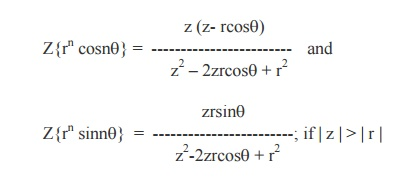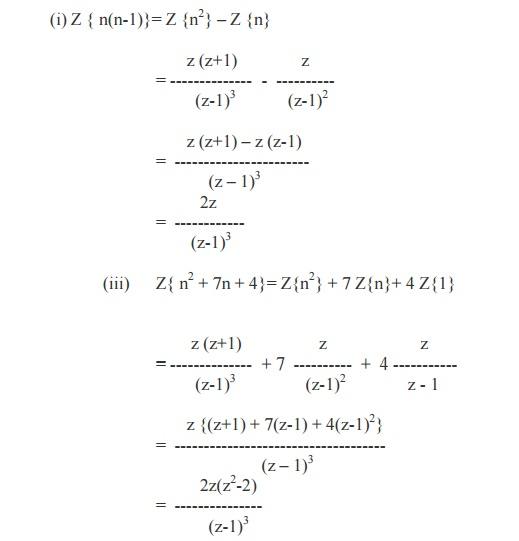Home | | Transforms and Partial Differential Equations | Z - Transforms and its Properties

# Z - Transforms and its Properties

Let {fn} be a sequence defined for n = 0,1,2,…….,then its Z-transform F(z) is defined as F(z)

Z - Transforms and its Properties

Definition

Let {fn} be a sequence defined for n = 0,1,2,…….,then its Z-transform F(z) is defined aswhenever the series converges and it depends on the sequence {fn}.

The inverse Z-transform of F(z) is given by Z-1{F(z)} = {fn}.

Note: If {fn}   is   defined   for   n   =   0,   ±   1,   ±   2,   …….,Properties of Z-Transforms

1.     The Z-transform is linear.

i.e,  if F(z) = Z{fn} and G(z) = Z{gn}, then

Z{afn + bgn} = aF(z) + bG(z).4. If Z{fn} = F(z), then

Z{fn+k}= zk{ F(z) –f0 –(f 1 / z ) - … - ( fk-1 / zk-1) } (k > 0)

ProofIn Particular,

(i) Z{f n+1} = z {F(z) - f0

(ii) Z{f n+2}= z2 { F(z) –f0 –(f1/z) }

Corollary

If  Z{fn} = F(z), then Z{fn–k} = z-k F(z).

(5) Initial value TheoremProof

We know that F (z) = f0 + f1 z-1 + f2z-2 + . . .

Taking limits as z ® ¥on both sides, we get(6) Final value TheoremSOME STANDARD RESULTS

1.                 Z{an} = z / (z-a), for |z| > |a|.

Proof

By definition, we haveIn particular, we have

Z{1} = z / (z-1), (taking a = 1).

and      Z{(-1)n} = z / (z +1), (taking a = -1).

2.  Z{nan} =  az /(z-a)2

Proof: By property, we haveSimilarly, we can prove

Z{n2an} = {az(z+a)}/ (z-a)3Equating the Real and Imaginary parts, we getEquating the Real and Imaginary parts, we getTable of Z –TransformsExample 2

Find the Z–transform of

1.     n(n-1)

2.     n2 + 7n + 4

3.     (1/2)( n+1)(n+2)Example 3

Find the Z- transforms of 1/n   and  1/n(n+1)= - log (1 –1/z ) if  |1/z| < 1

= - log (z-1 / z)

=   log (z/z-1), if | z | >1.Example 4

Find the Z- transforms of

(i) cos np/2

(ii)   sin np/2Example 5

Show that Z{1/ n!} = e1/z and hence find Z{1/ (n+1)!} and  Z{1/ (n+2)!}We know that Z{fn+1} = z { F(z) –f0}

Therefore,Example 6

Find the Z- transforms of the followingStudy Material, Lecturing Notes, Assignment, Reference, Wiki description explanation, brief detail
Mathematics (maths) : Z-Transforms and Difference Equations : Z - Transforms and its Properties |